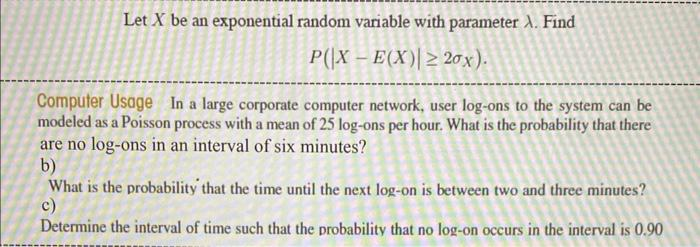# Question Solved1 AnswerPlease help Let $$X$$ be an exponential random variable with parameter $$\lambda$$. Find $P\left(|X-E(X)| \geq 2 \sigma_{X}\right) .$ Computer Usage In a large corporate computer network, user log-ons to the system can be modeled as a Poisson process with a mean of 25 log-ons per hour. What is the probability that there are no log-ons in an interval of six minutes? b) What is the probability that the time until the next log-on is between two and three minutes? c) Determine the interval of time such that the probability that no log-on occurs in the interval is $$0.90$$HXVS6O The Asker · Probability and StatisticsTranscribed Image Text: Let $$X$$ be an exponential random variable with parameter $$\lambda$$. Find $P\left(|X-E(X)| \geq 2 \sigma_{X}\right) .$ Computer Usage In a large corporate computer network, user log-ons to the system can be modeled as a Poisson process with a mean of 25 log-ons per hour. What is the probability that there are no log-ons in an interval of six minutes? b) What is the probability that the time until the next log-on is between two and three minutes? c) Determine the interval of time such that the probability that no log-on occurs in the interval is $$0.90$$
More
Transcribed Image Text: Let $$X$$ be an exponential random variable with parameter $$\lambda$$. Find $P\left(|X-E(X)| \geq 2 \sigma_{X}\right) .$ Computer Usage In a large corporate computer network, user log-ons to the system can be modeled as a Poisson process with a mean of 25 log-ons per hour. What is the probability that there are no log-ons in an interval of six minutes? b) What is the probability that the time until the next log-on is between two and three minutes? c) Determine the interval of time such that the probability that no log-on occurs in the interval is $$0.90$$